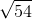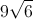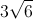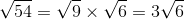# ISEE Middle Level Math : How to find the square root

## Example Questions

### Example Question #51 : Squares / Square Roots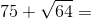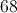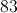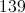Explanation:

First, find the square root: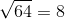Then, solve: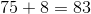### Example Question #52 : Squares / Square Roots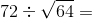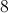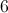Explanation:

First, find the square root:Then, solve: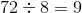### Example Question #53 : Squares / Square Roots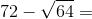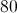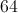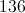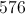Explanation:

First, find the square root:Then, solve: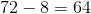### Example Question #54 : Squares / Square Roots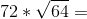576576

Explanation:

First, find the square root:Then, solve: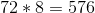### Example Question #55 : Squares / Square Roots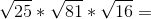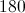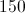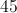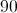Explanation:

First, find the sqaure root: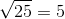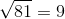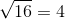Then, solve: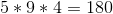### Example Question #56 : Squares / Square Roots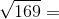Explanation:

Find the square root: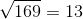### Example Question #57 : Squares / Square Roots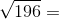Explanation:

Find the square root: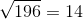### Example Question #58 : Squares / Square Roots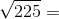Explanation:

Find the square root: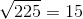### Example Question #59 : Squares / Square Roots

Evaluate: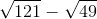Explanation: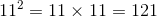, so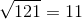.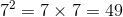,  so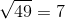.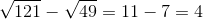### Example Question #60 : Squares / Square Roots

Simplify: